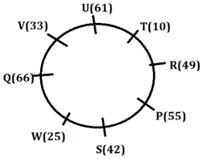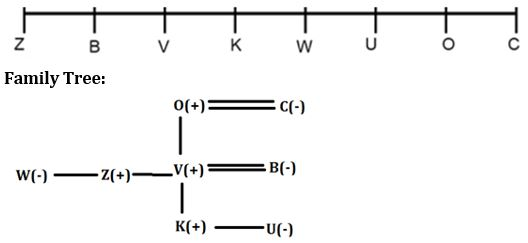Latest Banking jobs   »

# Reasoning Ability Quiz For IBPS RRB PO Clerk Mains 2022- 8th September

Direction (1-5): Study the following information carefully and answer the questions accordingly.
Eight people P, Q, R, S, T, U, V, and W are sitting around a circular table facing outside. Each one of them has a different number of books i.e. 10, 25, 33, 42, 49, 55, 61, and 66 but not necessarily in the same order.
Two people are sitting between S who is not an immediate neighbor of Q and the one who has 33 books. U is sitting immediate right of the one who has 33 books. Two people are sitting between the one who has a square number of books i.e., less than 42 and U. At most two people are sitting between the one who has a square number of books i.e., less than 42 and the one who has 33 books. The one who has the least number of books sits third to the right of Q who does not have 33 or 25 books. S does not have the least number of books. P who does not have 25 books sits immediate right of R who does not have the least number of books. T has an even number of books. The difference between T’s and V’s books is more than 15. At least one person is sitting between P and W. R has a square number of books. Q has an even number of books and more books than R has. P’s immediate neighbor has an even number of books. U has more books than P has.

Q1. Who has the least number of books?
(a) S
(b) W
(c) R
(d) T
(e) None of these

Q2. How many people sit between V and the one who has 42 books from the right of V?
(a) Four
(b) Five
(c) Two
(d) Six
(e) None of these

Q3. What is the position of U from the one who has the maximum number of books?
(a) Second to the right
(b) Third to the left
(c) Fourth to the left
(d) Immediate right
(e) None of these

Q4. Four from the following are similar in a certain way and forms a group. Find out the one who does not belong to that group.
(a) V
(b) R
(c) W
(d) T
(e) P

Q5. Who is sitting third to the right of R?
(a) V
(b) The one, who has 42 books
(c) Q
(d) The one who has 25 books
(e) None of these

Direction (6-10): Study the following information and answer the given questions.
There are eight members in a family – V, W, O, B, U, C, K, and Z. Each of them has a relationship with V – mother, sister, wife, son, father, brother and daughter but not necessarily in the same order. They are sitting in a single line facing North.
C is an immediate neighbour of V’s father. Two people are sitting between U and W’s brother. Two people sit between V’s son and V’s father. C is sitting at the right end of the line. Z’s father sits to the immediate right of V’s daughter. V is not an immediate neighbour of U. V’s wife is an immediate neighbour of Z. K is younger than O. V’s mother sits at one of the ends of the line. U sits second to the right of V’s son. B and V’s brother are sitting together. V’s brother sits second to the left of V. Two people are sitting between B and V’s sister.

Q6. How many people are sitting between U’s mother and V?
(a) One
(b) Two
(c) Four
(d) Three
(e) None

Q7. Who is V’s brother’s sister?
(a) O
(b) C
(c) Z
(d) U
(e) W

Q8. Who is V’s wife?
(a) B
(b) W
(c) C
(d) U
(e) Z

Q9. Who sits second to right of V’s sister?
(a) V
(b) C
(c) U
(d) O
(e) None of these

Q10. How many people are sitting between B and V’s daughter?
(a) Two
(b) Three
(c) Five
(d) Six
(e) One

Direction (11-13): In the following questions assuming the given statement to be true, find which of the conclusion(s) among given conclusions is/are definitely true and then give your answers accordingly.

Q11. Statements: F = A > B; C ≥ D; G < E ≤ D; C ≤ B; G=R >H
Conclusions: I. C ≥ R
II. A > G
III. F ≥ D
(a) Only I is true
(b) Only II is true
(c) Either I or III is true
(d) None is true
(e) Both II and III are true

Q12. Statements: R > C ≥M = N < T ≤ S; A ≥ B =D < N = J ≥P
Conclusions: I. P < S
II. A > T
III. R ≥ D
(a) Only I is true
(b) Only III is true
(c) Either I or II is true
(d) Both II and III are true
(e) Both I and II are true

Q13. Statements: O > M = P ≤ W < K; P > F ≥ U; S <U
Conclusions: I. O > U
II. M > K
III. S ≤ W
(a) Only I is true
(b) Only II is true
(c) Either II or III is true
(d) Only III is true
(e) Both I and II are true

Directions: (14-15): Answer the questions based on the information given below.
A % B means A is not smaller than B
A@B means A is neither smaller than nor equal to B
A & B means A is neither greater than nor equal to B
A\$B means A is not greater than B
A# B means A is neither smaller than nor greater than B

Q14. Statements: A%B#R@Q&S\$N, N%M@P\$O@L
Conclusion: I. A@Q
II. N@P
III. N & R
(a) Only Conclusion I follows
(b) Only Conclusion II follows
(c) Only Conclusion III follows
(d) Only Conclusions I and II follow
(e) Only Conclusions I and III follow

Q15. Statements: S%A#V&U&H\$N, P%Q#R\$O&J
Conclusions: I. J & P
II. A@N
III. Q & J
(a) Only Conclusion I follows
(b) Only Conclusion II follows
(c) Only Conclusion III follows
(d) Only Conclusions I and II follow
(e) Only Conclusions I and III follow

Solutions

Solution (1-5):
Sol.S1. Ans. (d)
S2. Ans. (a)
S3. Ans. (a)
S4. Ans. (d)
S5. Ans. (d)

Solution (6-10):
Sol.S6. Ans. (e)
S7. Ans. (e)
S8. Ans. (a)
S9. Ans. (d)
S10. Ans. (b)

S11. Ans. (b)
Sol. I. C ≥ R – False
II. A > G – True
III. F ≥ D – False

S12. Ans. (a)
Sol. I. P < S – True
II. A > T – False
III. R ≥ D – False

S13. Ans. (a)
Sol. I. O > U – True
II. M > K – False
III. S ≤ W – False

S14. Ans. (d)
Sol. I. A@Q – A>Q – True
II. N@P – N>P – True
III. N & R – N<R – False

S15. Ans. (c)
Sol. I. J & P – J < P – False
II. A@N – A>N – False
III. Q & J – Q <J – True#### Congratulations!Download Hindu Review of October 2021: Free PDF